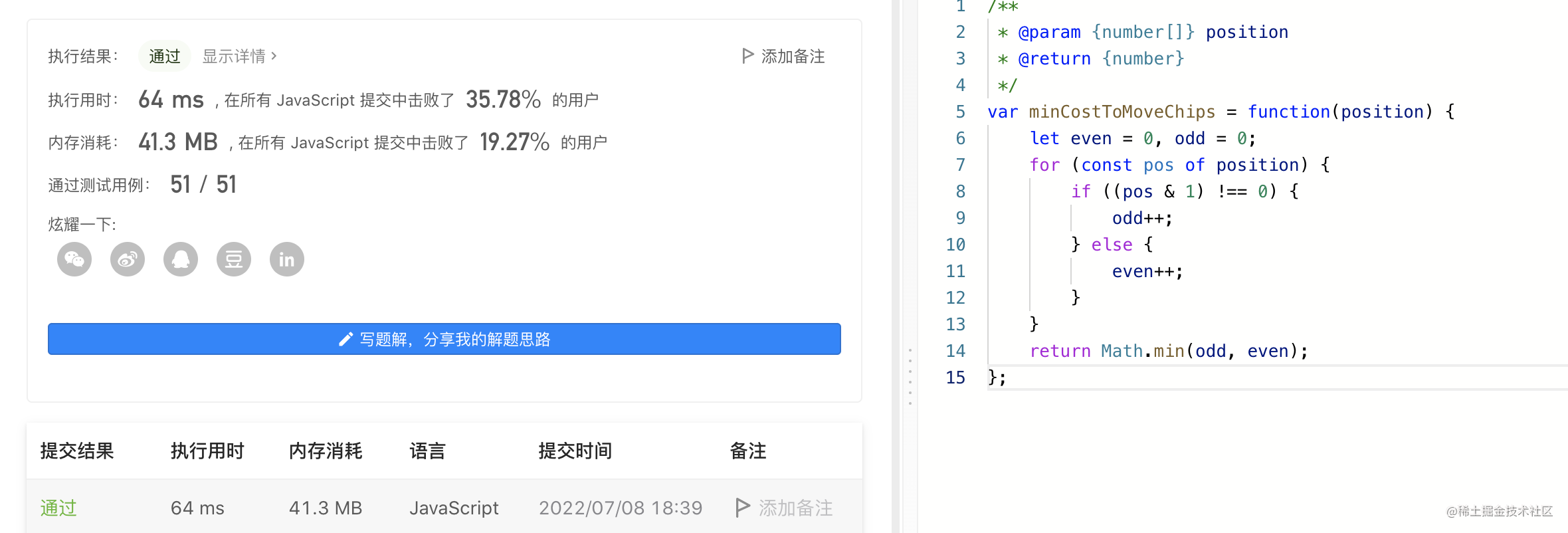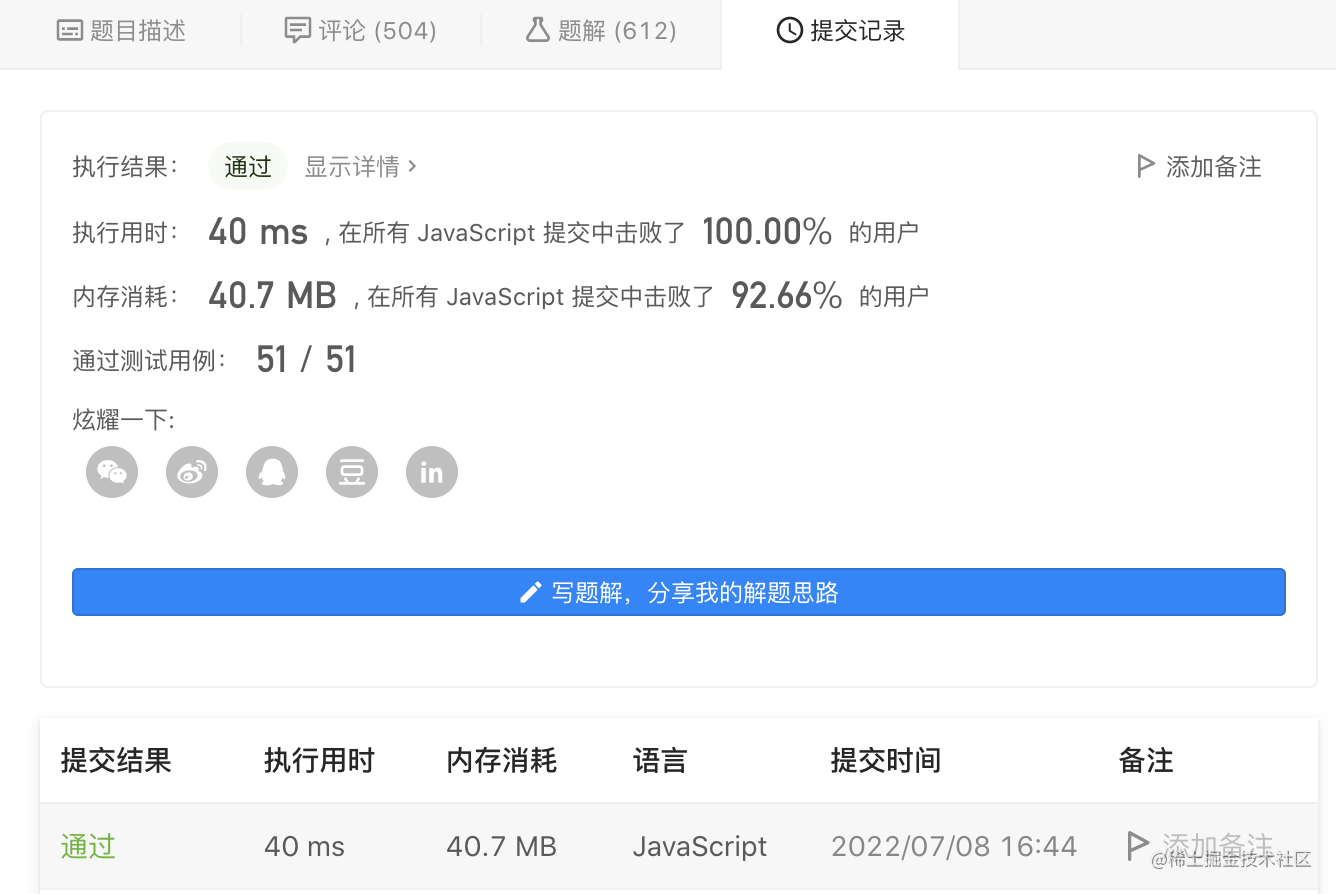# 1217. 玩筹码 javascript

## 题目描述

position[i] + 2 或 position[i] - 2 ，此时 cost = 0 position[i] + 1 或 position[i] - 1 ，此时 cost = 1 返回将所有筹码移动到同一位置上所需要的 最小代价 。

1 <= chips.length <= 100 1 <= chips[i] <= 10^9

## 解题思路

``````position[i] + 2 或 position[i] - 2 ，此时 cost = 0
position[i] + 1 或 position[i] - 1 ，此时 cost = 1

## 官方解法

``````/**
* @param {number[]} position
* @return {number}
*/
var minCostToMoveChips = function(position) {
let even = 0, odd = 0;
for (const pos of position) {
if ((pos & 1) !== 0) {
odd++;
} else {
even++;
}
}
return Math.min(odd, even);
};## 自己解法

``````/**
* @param {number[]} position
* @return {number}
*/
var minCostToMoveChips = function(position) {
let odd = 0 ;
let even = 0 ;
for( let i = 0; i < position.length; i++){
if(position[i]%2==0){
even++;
}else{
odd++;
}
}
return even>odd?odd:even;
};